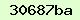# Decibels in the context of ADSL

## The decibel

The decibel is a logarithmic unit which specifies the ratio of two powers. It was devised by the Bell Telephone Company to simplify calculations of gain and loss in cascaded transmission systems. The original unit was the Bel, and they defined this as:

```Number of Bels = logarithm (base 10) of the ratio OutputPower / InputPower.

```

Because this was rather too coarse a unit for many purposes, they specified the decibel (dB) as one tenth of a Bel. This may seem a rather cumbersome way of specifying a power gain or loss, but it has some advantages:

1. Because of the way that logarithms work, the overall gain of several cascaded stages is simply the sum of the decibel gains of each stage. This is a lot easier than multiplying and dividing the power ratios.

2. The log of a number less than 1 is negative, so all power gains have positive dB values and all losses have negative dB values. If the input and output powers are equal, then the gain is 0 dB.

Some example values

 Decibel value Arithmetic equivalent -6 dB 0.25 -3 dB 0.5 0 dB 1 3 dB 2 6 dB 4 10 dB 10 20 dB 100 30 dB 1000

### Attenuation

Ordinary twisted-pair telephone lines were never designed for the high frequencies used in ADSL transmission. Not surprisingly a lot of the ADSL signal gets lost between the exchange and the user. What may surprise you is just how much gets lost...

Prior to 6 September 2004, the limiting attenuation figure for a UK ADSL installation was 60 dB (i.e. a gain of -60 dB). This is equal to 6 Bels, and therefore the logarithm of the power ratio is 6. In other words, the signal at the end of the line is just 10-6 (one-millionth) of the signal transmitted from the exchange. And as from 6 September 2004 the limits were relaxed further, with 75 dB being the level of attenuation at which a usable ADSL service is considered extremely unlikely - that's a power ratio of about 32 million. Lower levels of attenuation are obviously preferable.

### Signal-to-noise ratio

Just to make life even more complicated for the receiving equipment, spurious signals get induced into the lines carrying the ADSL signal. These spurious signals can come from other telephone lines running alongside, from external electrical sources, from poor quality connections, and from electronic circuits processing the signals. Together, these unwanted signals are referred to as 'noise'. Clearly, to enable the receiving equipment to separate the wanted signal from the noise, the wanted signal must be greater than the noise by a reasonable margin. The ratio between the wanted and unwanted signals is called the signal-to-noise ratio, or SNR. The greater this ratio is, the better.

The limiting value of SNR is generally taken to be 6 dB (= 0.6 Bel). This corresponds with a power ratio of 4 (log 4 = 0.6). So the wanted signal power must be at least four time the noise power. And because power is proportional to the square of the voltage, this means that the signal voltage must be at least double the noise voltage. Quite obviously, any lower values of SNR will make it very difficult to separate out a clean ADSL signal.

It should be noted that ADSL modems and routers generally report noise margin (or SNR margin), not SNR. The manufacturer specifies a SNR at which the error count reaches some threshold beyond which the connection would not be acceptably stable; the noise margin is the amount by which the measured SNR exceeds this base level of SNR. So, for example, if the minimum acceptable SNR was set at 6 dB, and the measured SNR was 10 dB, then the modem/router would report a noise margin of 4 dB.

### Decibels used as power measurement

As described above, decibels specify a ratio between two numbers (one number divided by the other). An extension to this is the dBm, which is defined as dB referenced to 1 mW (one milliwatt). This is a convenient way to specify a wide range of power levels in fairly small numbers. So the ratio of the actual power of a device to 1 mW is converted to dB, and then the power is specified as that number of dBm. For example:

 Power dBm equivalent 1 mW 0 dBm 2 mW 3 dBm 10 mW 10 dBm 100 mW 20 dBm 1 W (1000 mW) 30 dBm 100 W (100,000 mW) 50 dBm

Most modem/routers report the upstream and downstream power levels in dBm, although in some cases this information is only available by using the telnet interface. The upstream (or local) power is the power transmitted by your modem/router, and the downstream (or remote) power is the power transmitted by the DSLAM or MSAN in the exchange.

0 (0)
Attached Files
Name
Email
Security CodeWireshark Trace for Fault Finding
Viewed 6766 times since Tue, Jul 25, 2017
I can’t connect to the Internet
Viewed 1999 times since Thu, Jul 27, 2017
Viewed 2509 times since Wed, Jul 26, 2017
Viewed 2302 times since Wed, Jul 26, 2017
PPPoE Discovery Stages
Viewed 6893 times since Mon, Aug 7, 2017